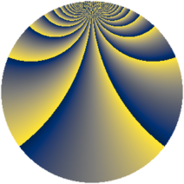# Properties

 Label 2240.2.ezLevel $2240$ Weight $2$ Character orbit 2240.ez Rep. character $\chi_{2240}(107,\cdot)$ Character field $\Q(\zeta_{48})$ Dimension $6080$ Sturm bound $768$

# Related objects

## Defining parameters

 Level: $$N$$ $$=$$ $$2240 = 2^{6} \cdot 5 \cdot 7$$ Weight: $$k$$ $$=$$ $$2$$ Character orbit: $$[\chi]$$ $$=$$ 2240.ez (of order $$48$$ and degree $$16$$) Character conductor: $$\operatorname{cond}(\chi)$$ $$=$$ $$2240$$ Character field: $$\Q(\zeta_{48})$$ Sturm bound: $$768$$

## Dimensions

The following table gives the dimensions of various subspaces of $$M_{2}(2240, [\chi])$$.

Total New Old
Modular forms 6208 6208 0
Cusp forms 6080 6080 0
Eisenstein series 128 128 0

## Trace form

 $$6080q - 8q^{2} - 8q^{3} - 8q^{5} - 64q^{6} - 16q^{7} - 32q^{8} + O(q^{10})$$ $$6080q - 8q^{2} - 8q^{3} - 8q^{5} - 64q^{6} - 16q^{7} - 32q^{8} - 8q^{10} - 16q^{11} - 8q^{12} - 32q^{13} - 64q^{14} - 32q^{15} - 16q^{16} - 16q^{17} - 8q^{18} - 32q^{20} - 32q^{21} - 32q^{22} - 8q^{23} - 8q^{25} - 16q^{26} - 32q^{27} - 16q^{28} + 56q^{30} - 32q^{31} - 8q^{32} + 64q^{34} - 16q^{35} - 64q^{36} - 8q^{37} - 8q^{38} - 136q^{40} - 64q^{41} - 16q^{42} - 32q^{43} + 16q^{45} - 16q^{46} - 32q^{48} - 32q^{50} - 16q^{51} - 8q^{52} - 8q^{53} - 32q^{55} - 32q^{56} - 32q^{57} + 56q^{58} - 8q^{60} - 16q^{61} - 16q^{65} + 80q^{66} - 8q^{67} + 56q^{68} - 16q^{70} + 64q^{71} - 8q^{72} - 8q^{73} - 8q^{75} - 64q^{76} - 16q^{77} - 336q^{78} - 8q^{80} - 16q^{81} - 88q^{82} - 32q^{83} + 48q^{85} - 16q^{86} - 8q^{87} - 96q^{88} - 32q^{90} - 32q^{91} - 176q^{92} + 40q^{93} - 64q^{94} - 16q^{95} - 16q^{96} - 56q^{98} + O(q^{100})$$

## Decomposition of $$S_{2}^{\mathrm{new}}(2240, [\chi])$$ into newform subspaces

The newforms in this space have not yet been added to the LMFDB.